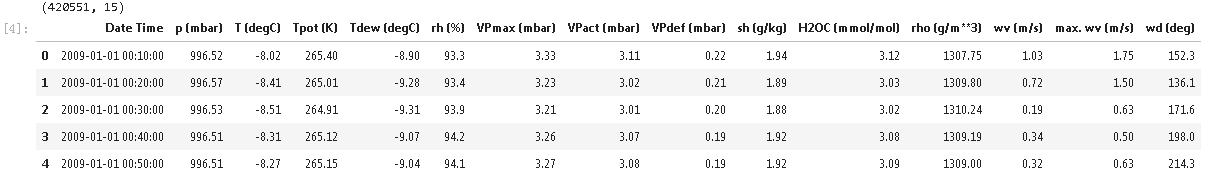# Prophet异常检测使用了Prophet时间序列预测。基本的Prophet模型是一个可分解的单变量时间序列模型，结合了趋势、季节性和节假日效应。

## 数据集

import matplotlib.pyplot as plt
import numpy as np
import os
import pandas as pd
import tensorflow as tf

## 加载数据集

df = pd.read\_csv(csv\_path)
df$'Date Time'$ = pd.to_datetime(df$'Date Time'$, format='%d.%m.%Y %H:%M:%S')
df.head()选择子集来测试Prophet模型。




n_prophet = 10000

Prophet模型需要得到一个有两列的DataFrame：一列名为ds，包含时间戳，一列名为y，包含要评估的时间序列。我们只看温度数据。

print(df_T.shape)
df_T.head()

plt.plot(df\_T$'ds'$, df\_T$'y'$)

## 加载或定义离群检测

filepath = 'my_path'  # 改为下载模型的目录
if outlier_detector:  # 加载预训练的离群检测器
filepath = os.path.join(filepath, detector_name)
else:  # 初始化、拟合并保存离群检测
od.fit(df_T)

# Prophet在R语言中进行时间序列数据预测

## 预测测试数据中的异常值

df\_T\_test = pd.DataFrame(data=d)

### 随时关注您喜欢的主题

df_T.tail()

predict(
df\_T\_test
)

## 结果可视化

model.predict(future)
model.plot(forecast)

# 我们还可以绘制预测中不同成分的细分。预测的不确定性区间是由外推趋势的MAP估计值决定的。

plot_component(forecast)

# 让我们把实际数据与离群点阈值的上限和下限预测值叠加起来，检查我们预测的离群点在哪里。

plot(x='ds', y=$'y', 'yhat', 'yhat\_upper', 'yhat\_lower'$)

np.zeros(n_periods)
plot(x='ds', y=$'score', 'threshold'$)

outlier = fcst.loc$fcst\['score'$ > 0\]
print((outlier.shape$0$))

Kaizong Ye拓端研究室（TRL）的研究员。

​非常感谢您阅读本文，如需帮助请联系我们！QQ在线咨询

15121130882

0571-63341498

## 关注有关新文章的微信公众号

This will close in 0 seconds